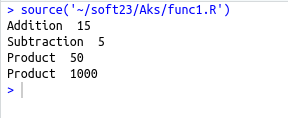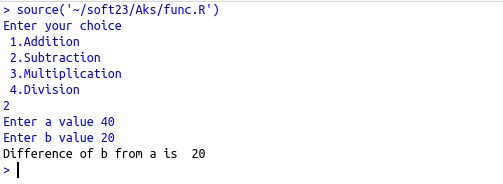• #5, First Floor, 4th Street Dr. Subbarayan Nagar Kodambakkam, Chennai-600 024 Landmark : Samiyar Madam
• pro@slogix.in
• +91- 81240 01111

### What is Functions in R?

###### Description

To understand the functions available in R,how to define a function and call the same.

###### Process
• Functions are block of codes that performs a specified task.Types of functions available in R User defined functions
• Built-in functions

User defined functions :
Syntax :

Function_name<-function(arg1,arg2,…)

{

Function body

Return()

}

Function arguments and return value :

• Function may or may not return a value
• Function may or may not have arguments
• Function can also have default arguments
###### Sapmle Code

Source code :(User-defined functions)

{
sum=a+b
cat(“Sum of a and b is “,sum)
}
sub<-function(a,b)
{
diff=a-b
cat(“Difference of b from a is “,diff)
}
mul<-function(a,b)
{
product=a*b
cat(“Product of a and b is “,product)
}
div<-function(a,b)
{
quo=a/b
cat(“Quotient from a/b is “,quo)
}
a=as.integer(readline(prompt=”Enter a value “))
b=as.integer(readline(prompt=”Enter b value “))

Source code :(Function arguments and return values)

{
a=5
b=10
c=a+b
return(c) #Function with return value
}
sub<-function(a,b)
{
c=a-b
return(c)
}
mul<-function(a=5,b=10)
{
return(a*b)
}